### Differentiation and Integration of Trigonometric functions

A lot of students confuse the differentiation of trig functions with the integration of trig functions

The most effective way is to study them togetherRemember that: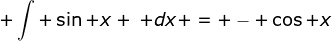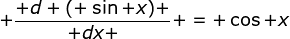We can differentiate tan x but we cannot integrate tan x

Example 1FindExample 2Note thatExample 3Find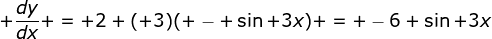Example 4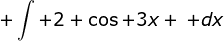Note that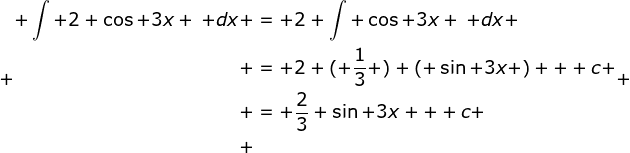1.You can integrate tanx however. You allow tanx to equal sinx/cosx, and that will integrate to -1ln|cosx| which equals ln|secx|+c.

2.Thank you - it helped a lot!

3.Each of the trigonometric capacities has a valuable reverse. In this way, for occasion, the reverse of the sine capacity is known as the arcsine, the opposite of the cosine is known as the arccosine, and the converse of the digression is known as the arctangent. http://www.mordocrosswords.com/2016/02/trigonometric-function_23.html